## Wednesday, July 26, 2017

### New phytools version submitted to CRAN

I'm submitting a new version of phytools to CRAN. The last update went up around the end of March, so needless to say the package has seen many fixes, modifications, and even a number of new functions since that time. Here is a non-comprehensive list - but all changes to phytools can also be tracked on its GitHub page.

1. New function to add tip labels to the tree with linking lines (here).

2. Update to the function `treeSlice` to crop the tipward end of a phylogeny (here).

3. New S3 `print` method for `evolvcv.lite` (here).

4. A fix to the S3 `plot` method for `"phyl.RMA"` class objects (here).

5. A significant extension of the S3 `density` method for `"multiSimmap"` class objects (here).

6. Fix & extension of the S3 method `plot.changesMap` to visualize all the changes from a set of stochastic character map trees (here).

7. Update to `plot.cophylo` for co-phylogenetic plotting to permit different edge colors to be used in one or the other plotted trees (here).

8. A completely new method, `ratebytree`, for comparing the evolutionary rate for a continuous character between two or more trees (here).

9. A complete re-write of phytools `densityTree` function for plotting a sample of trees, including with mapped discrete characters (here).

10. An update to the `plot` method for the `"simmap"` and `"multiSimmap"` object classes to permit slanted phylograms (here).

11. A completely new function to compare the node heights of two plotted chronograms (aka. time-trees; here).

12. A simple new function, `geo.legend`, to overlay a geological timescale onto a plotted tree (here).

13. A general function and some associated methods for expanding or contracting the tip spacing of certain subtrees on a plotted phylogeny (here).

14. A small bug fix in the popular function `fastBM` for non 'cladewise' ordered trees (here).

15. Another bug fix for `read.newick` for the cases in which some but not all internal nodes are labeled (here).

16. New user control over the color & width of the plotted arcs in `arc.cladelabels` (here).

The CRAN submission has version number 0.6-20. Hopefully it is accepted. I'll keep you posted! It is already available via GitHub.

## Wednesday, July 12, 2017

### Fitting discrete character (Mk) models with a fixed state at the root

“…how do I specify an ancestral state for my root node phytools?? And can I specify one when using the ER model of character evolution? I feel this should be really simple to answer, sorry but if you could direct me to an answer that would be fantastic.”

I'm going to interpret this as meaning “how do I fit a discrete character model in which the state at the global root is known.”

This is not super easy. It is most straightforward to do using `fitMk`, but can also be accomplished using `fitDiscrete` of the geiger package.

First I'll demonstrate `fitMk`.

Here are our tree & data:

``````library(phytools)
library(RColorBrewer)
states<-sort(unique(x))
plotTree(tree,type="fan",ftype="off")
tiplabels(tip=1:Ntip(tree),pie=to.matrix(x,states),
piecol=brewer.pal(3,"Accent"),cex=0.6)
states),shape="circle",prompt=FALSE,x=-1,y=-0.8)
``````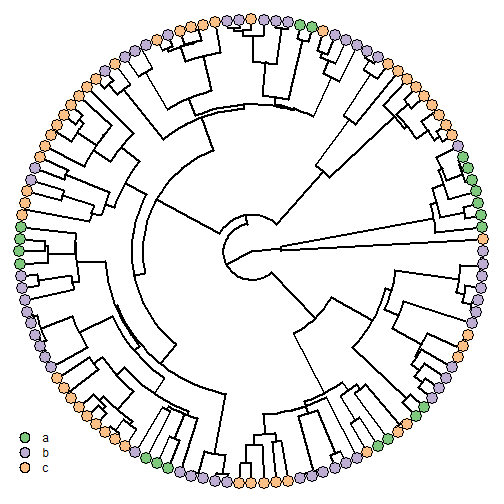Now, we're going to use an 'ordered' model - but in which we either use a flat prior probability distribution on the global root, or we fix the root to each of the three possible states:

``````model<-matrix(c(0,1,0,1,0,1,0,1,0),3,3,dimnames=list(states,
states))
model
``````
``````##   a b c
## a 0 1 0
## b 1 0 1
## c 0 1 0
``````
``````fit.flat<-fitMk(tree,x,model=model)
fit.flat
``````
``````## Object of class "fitMk".
##
## Fitted (or set) value of Q:
##           a         b         c
## a -1.204273  1.204273  0.000000
## b  1.204273 -2.408547  1.204273
## c  0.000000  1.204273 -1.204273
##
## Fitted (or set) value of pi:
##         a         b         c
## 0.3333333 0.3333333 0.3333333
##
## Log-likelihood: -79.285705
##
## Optimization method used was "nlminb"
``````
``````fit.a<-fitMk(tree,x,model=model,pi=setNames(c(1,0,0),states))
fit.a
``````
``````## Object of class "fitMk".
##
## Fitted (or set) value of Q:
##           a         b         c
## a -1.266891  1.266891  0.000000
## b  1.266891 -2.533781  1.266891
## c  0.000000  1.266891 -1.266891
##
## Fitted (or set) value of pi:
## a b c
## 1 0 0
##
## Log-likelihood: -80.385615
##
## Optimization method used was "nlminb"
``````
``````fit.b<-fitMk(tree,x,model=model,pi=setNames(c(0,1,0),states))
fit.b
``````
``````## Object of class "fitMk".
##
## Fitted (or set) value of Q:
##           a         b         c
## a -1.148579  1.148579  0.000000
## b  1.148579 -2.297158  1.148579
## c  0.000000  1.148579 -1.148579
##
## Fitted (or set) value of pi:
## a b c
## 0 1 0
##
## Log-likelihood: -78.905018
##
## Optimization method used was "nlminb"
``````
``````fit.c<-fitMk(tree,x,model=model,pi=setNames(c(0,0,1),states))
fit.c
``````
``````## Object of class "fitMk".
##
## Fitted (or set) value of Q:
##           a         b         c
## a -1.251647  1.251647  0.000000
## b  1.251647 -2.503294  1.251647
## c  0.000000  1.251647 -1.251647
##
## Fitted (or set) value of pi:
## a b c
## 0 0 1
##
## Log-likelihood: -79.059712
##
## Optimization method used was "nlminb"
``````

Note that although one might be tempted to compare the likelihoods of these three fitted models, this should not in fact be done. This is because the likelihoods are in each case conditioned on the state at the root state being known - in other words, they are conditioned on different data. That this is not equivalent to constraining a particular parameter to have some value is most evident by comparison between the model in which the root is fixed to state `"b"` to the model in which the root state is unknown. If the former was a special case of the latter, then it should have a lower (or equal) likelihood, when in fact its likelihood is slightly higher. How come? Well in reality the fixed state likelihood is the probability that all the tips are in their observed states and that the root is in state `"b"`, given the tree, a model, and the model parameters (to be estimated); whereas the 'flat prior' model likelihood is simply the probability of the data at the tips, given the tree & model, but integrating over all possible values for the root node of the phylogeny.

We can do the same analysis using `fitDiscrete`, but it is not quite as straightforward. First, we have to use the method to generate a likelihood function, and then we can optimize that function using different prior probability distributions for the root of the tree, as follows:

``````library(geiger)
obj<-fitDiscrete(tree,x,model=model)
``````
``````## Warning in fitDiscrete(tree, x, model = model): Parameter estimates appear at bounds:
##  q13
##  q31
``````
``````obj
``````
``````## GEIGER-fitted comparative model of discrete data
##  fitted Q matrix:
##               a         b         c
##     a -1.184179  1.184179  0.000000
##     b  1.184179 -2.368358  1.184179
##     c  0.000000  1.184179 -1.184179
##
##  model summary:
##  log-likelihood = -79.073420
##  AIC = 160.146839
##  AICc = 160.180738
##  free parameters = 1
##
## Convergence diagnostics:
##  optimization iterations = 100
##  failed iterations = 0
##  frequency of best fit = 1.00
##
##  object summary:
##  'lik' -- likelihood function
##  'bnd' -- bounds for likelihood search
##  'res' -- optimization iteration summary
##  'opt' -- maximum likelihood parameter estimates
``````

(Note that `fitDiscrete` does not use a flat prior by default.)

``````lik<-obj\$lik
lik
``````
``````## likelihood function for univariate discrete trait evolution
##  argument names: q12
##
##  mapping
##      1: a
##      2: b
##      3: c
##
## definition:
## function (pars, ...)
## {
##     if (missing(pars))
##         stop(paste("The following 'pars' are expected:\n\t",
##             paste(argn(tmp), collapse = "\n\t", sep = ""), sep = ""))
##     pars = .repars(pars, argn(tmp))
##     tmp(pars, ...)
## }
## <bytecode: 0x00000000086ba470>
## <environment: 0x000000000bcfb828>
``````
``````fit.flat<-optimize(lik,c(1e-8,1000),root="flat",maximum=TRUE)
fit.flat
``````
``````## \$maximum
##  1.20427
##
## \$objective
##  -79.2857
``````
``````fit.a<-optimize(lik,c(1e-8,1000),root="given",
root.p=setNames(c(1,0,0),states),maximum=TRUE)
fit.a
``````
``````## \$maximum
##  1.266875
##
## \$objective
##  -80.38561
``````
``````fit.b<-optimize(lik,c(1e-8,1000),root="given",
root.p=setNames(c(0,1,0),states),maximum=TRUE)
fit.b
``````
``````## \$maximum
##  1.148578
##
## \$objective
##  -78.90502
``````
``````fit.c<-optimize(lik,c(1e-8,1000),root="given",
root.p=setNames(c(0,0,1),states),maximum=TRUE)
fit.c
``````
``````## \$maximum
##  1.251644
##
## \$objective
##  -79.05971
``````

For each fitted model, `\$maximum` is the single fitted model parameter; whereas `\$objective` is the log-likelihood. If we had a model with more than one parameter, we could use `optim` or some other numerical optimizer to fit the model.

Finally, don't forget that phytools has S3 plotting methods for both `fitMk` & `fitDiscrete`. E.g.:

``````plot(fit.phytools<-fitMk(tree,x,model="ARD"))
title(main="Result from phytools::fitMk(...,model=\"ARD\")")
title(main=paste("\n\n\nlog(L) =",round(logLik(fit.phytools),5)),
cex.main=1)
``````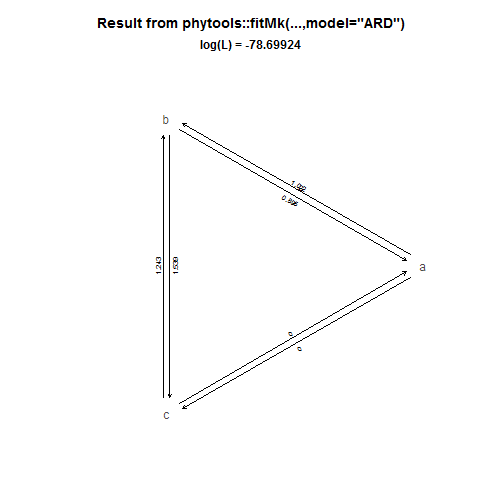``````plot(fit.geiger<-fitDiscrete(tree,x,model="ARD"))
title(main="Result from geiger::fitDiscrete(...,model=\"ARD\")")
title(main=paste("\n\n\nlog(L) =",round(logLik(fit.geiger),5)),
cex.main=1)
``````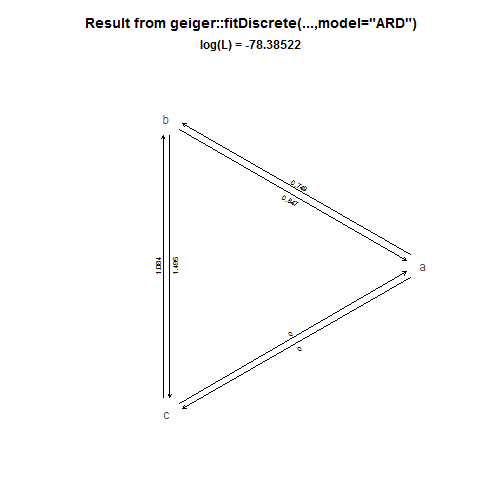(Note that these two fitted models, and their likelihoods, come out as different - but in reality the likelihoods are not directly comparable because the two functions make different assumptions about the prior on the root. If we force geiger's `fitDiscrete` likelihood function to use a flat prior, we should find that it yields a worse likelihood then we obtained using `phytools:::fitMk` - if, in fact, `fitMk` has converged on the MLE, e.g.:

``````lik<-fit.geiger\$lik
q<-fit.phytools\$rates
## fitMk paramater estimates
lik(pars=c(q12=q,q13=q,q21=q,q23=q,q31=q,q32=q),
root="flat")
``````
``````##  -78.69924
``````
``````## fitDiscrete estimates, but with flat root prior
lik(pars=unlist(fit.geiger\$opt[1:6]),root="flat")
``````
``````##  -78.77036
``````

It would also have been plausible that `fitMk` had failed to converge on the ML solution as, in general, the optimization routines used by `fitDiscrete` are more robust. This doesn't seem to the case in this instance.)

The data for this exercise were simulated as follows:

``````tree<-pbtree(n=120,scale=1)
Q<-matrix(c(-1,1,0,1,-2,1,0,1,-1),3,3,dimnames=list(letters[1:3],
letters[1:3]))
x<-as.factor(sim.history(tree,Q,anc="b")\$states)
``````

That's it.

## Friday, July 7, 2017

### Slides for Evolution 2017: A phytools plotting demo

The following is a demo of the phytools functions & methods I used to create the graphics for my Evolution 2017 meeting talk entitled “Some additional new methods for plotting phylogenies & comparative data.” The final slides for this talk are available here.

``````library(phytools)
packageVersion("phytools")
``````
``````##  '0.6.18'
``````
``````## -- Read some data from file:

## -- These methods simply plot the data at the tips of the tree. There also
## -- exists a number of other comparable functions.

dotTree(tree,x,length=10,ftype="i")
``````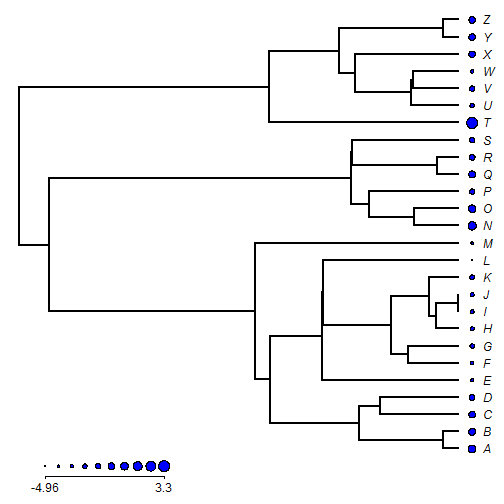``````plotTree.barplot(tree,x)
``````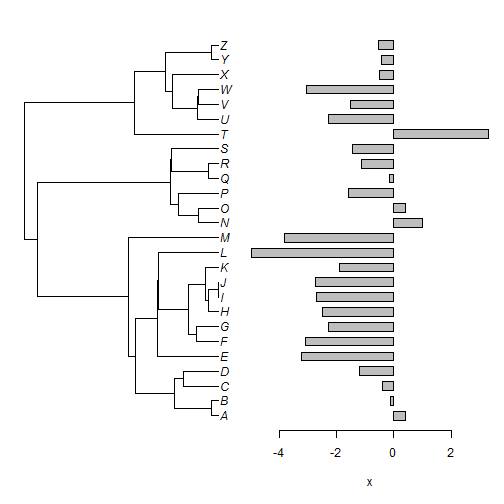``````plotTree.barplot(tree,x,args.barplot=list(col=sapply(x,
function(x) if(x>=0) "blue" else "red"),
xlim=c(-4,4)))
``````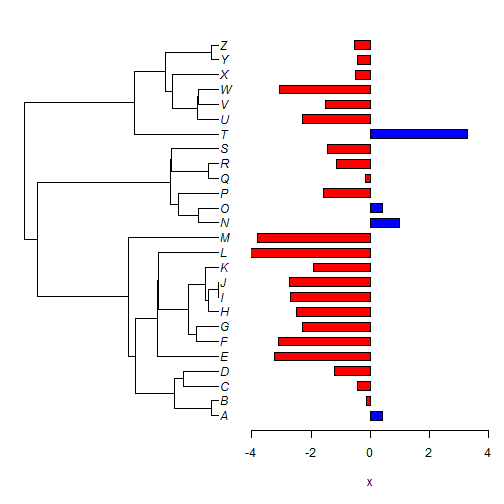``````## -- We can also plot the data at the tips of the tree for multiple traits
## -- simultaneously. For instance:

dotTree(tree,X,standardize=TRUE,length=10)
``````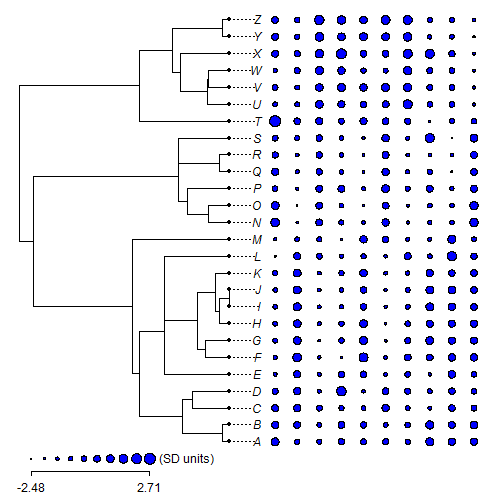``````phylo.heatmap(tree,X,standardize=TRUE)
``````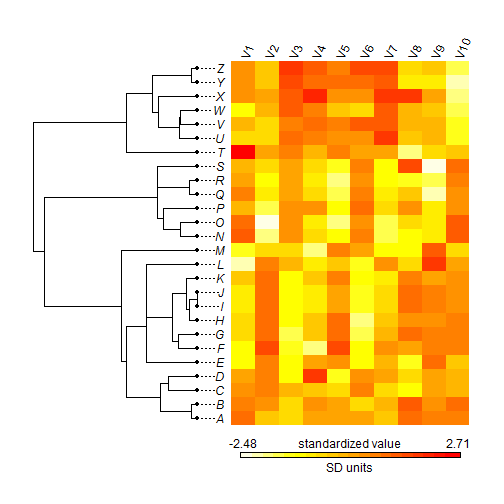``````## -- Aside from plotting the data at the tips, we can also visualize its
## -- reconstructed evolution. A simple method for doing this is called a
## -- 'traitgram' in which the phylogeny is projected into phenotype space
## -- as follows:

``````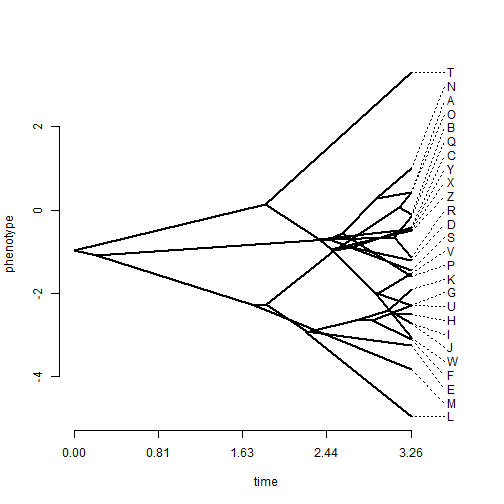``````## -- A variant of this function exists where the idea is to visualize the
## -- uncertainty around the reconstructed values at internal nodes, as
## -- follows:

``````
``````## Computing density traitgram...
``````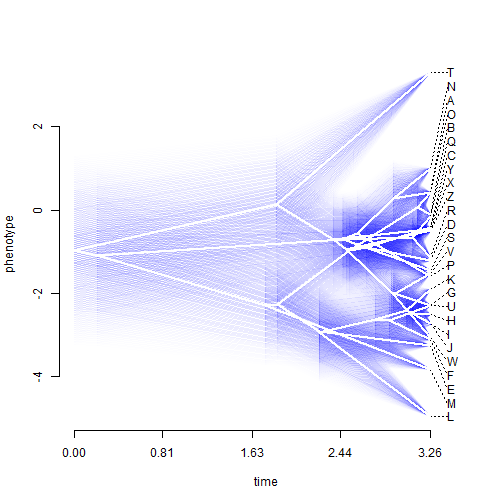``````## -- Another method in phytools projects reconstructed states onto internal
## -- edges & nodes of the tree using a color gradient. I will also demonstrate
## -- a helper function called errorbar.contMap which computes & adds error
## -- bars to the tree using the same color gradient as was employed in the
## -- plot.

obj<-contMap(tree,x,plot=FALSE)
plot(obj,lwd=7,xlim=c(-0.2,3.6))
errorbar.contMap(obj)
``````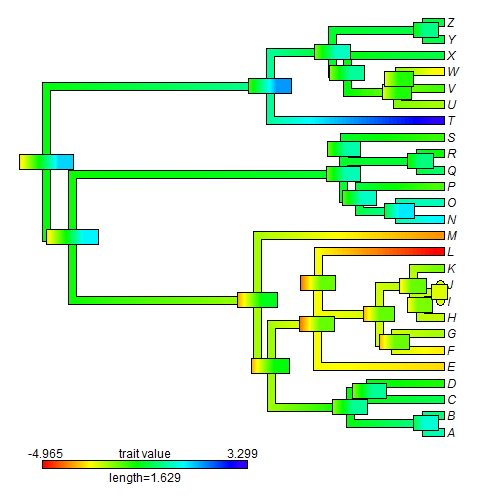``````## -- This method creates a special object class that can then be replotted
## -- without needing to repeat all the computation. We can also replot this
## -- object using different parameters. For example, in the following script
## -- I will invert & then gray-scale the color gradient of our original plot
## -- & then replot these two trees in a facing manner.

obj
``````
``````## Object of class "contMap" containing:
##
## (1) A phylogenetic tree with 26 tips and 25 internal nodes.
##
## (2) A mapped continuous trait on the range (-4.964627, 3.299418).
``````
``````obj<-setMap(obj,invert=TRUE)
grey<-setMap(obj,c("white","black"))
par(mfrow=c(1,2))
plot(obj,lwd=7,ftype="off",xlim=c(-0.2,3.6),legend=2)
plot(grey,lwd=7,direction="leftwards",ftype="off",xlim=c(-0.2,3.6),
legend=2)
``````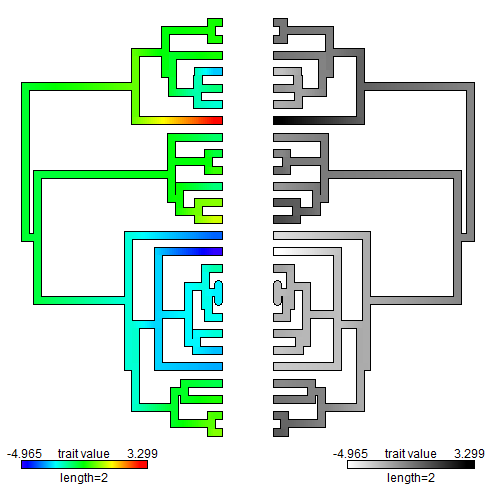``````## -- Now let's try the same thing but with some empirical data:

anole.tree
``````
``````##
## Phylogenetic tree with 100 tips and 99 internal nodes.
##
## Tip labels:
##  ahli, allogus, rubribarbus, imias, sagrei, bremeri, ...
##
## Rooted; includes branch lengths.
``````
``````svl<-read.csv("svl.csv",header=TRUE,row.names=1)
svl<-setNames(svl[,1],rownames(svl))
obj<-contMap(anole.tree,svl,plot=FALSE)
obj<-setMap(obj,invert=TRUE)
plot(obj,fsize=c(0.4,1),outline=FALSE,lwd=c(3,7),leg.txt="log(SVL)")
``````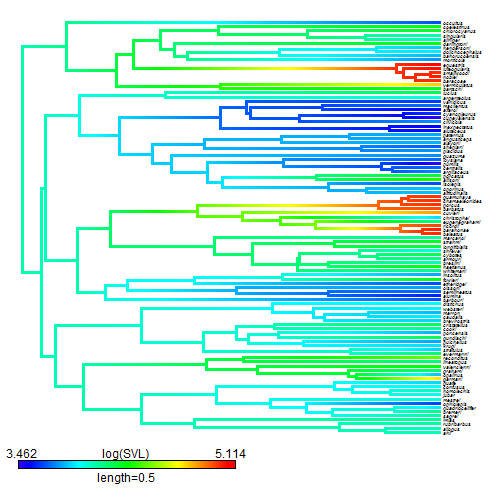``````## -- This plotting method is fairly flexible, as we've seen. We can also
## -- plot our tree in a circular or "fan" style. In the following demo,
## -- I will also show how to add raster images of some of the taxa to
## -- our plot, which is kind of cool.

plot(obj,type="fan",outline=FALSE,fsize=c(0.6,1),legend=1,
lwd=c(4,8),xlim=c(-1.8,1.8),leg.txt="log(SVL)")
spp<-c("barbatus","porcatus","cristatellus","equestris","sagrei")
library(png)
w<-0.5
for(i in 1:length(spp)){
arrl<-if(spp[i]%in%c("sagrei","porcatus")) 0.24
else if(spp[i]=="barbatus") 0.2 else 0.34
asp<-dim(img)/dim(img)
rasterImage(img,xy\$x0-w/2,xy\$y0-w/2*asp,xy\$x0+w/2,xy\$y0+w/2*asp)
}
``````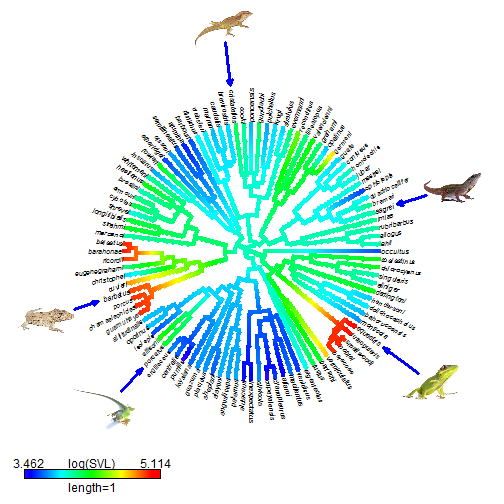``````## -- Another popular visualization method in phytools is something called a
## -- phylomorphospace, which is just a projection of the tree into (normally)
## -- a two-dimensional morphospace. For example:

phylomorphospace(tree,X[,1:2],xlab="trait 1",ylab="trait 2")
``````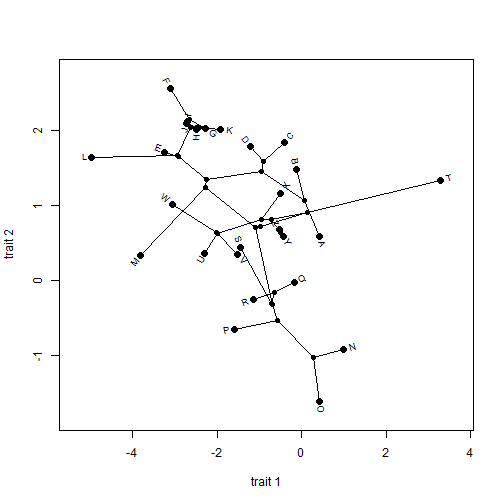``````## -- For more than three continuous trait dimensions phytools also has a method
## -- that I really like, although I've literally never seen it used in
## -- publication. I call this method a 'phylogenetic scatterplot matrix'
## -- because it contains unidimensional contMap plots on the diagonal &
## -- bivariate phylomorphospaces for each pair of traits in off-diagonal
## -- positions. This is what that looks like for five traits:

obj<-fancyTree(tree,type="scattergram",X=X[,1:5])
``````
``````## Computing multidimensional phylogenetic scatterplot matrix...
``````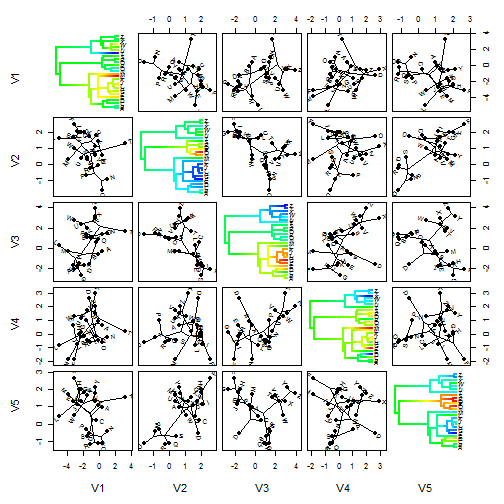``````## -- In addition to these continuous character methods, phytools also has a
## -- number of discrete character visualization tools for plotting discrete
## -- valued traits & trees.

## -- For instance, phytools contains a range of different methods related
## -- to a statistical approach called stochastic character mapping, in which
## -- dicrete character histories are sampled from their posterior probability
## -- distribution. For starters, I will just use a stochastic character map
## -- tree that can be loaded from the package.

data(anoletree)
cols<-setNames(palette()[1:6],mapped.states(anoletree))
plot(anoletree,cols,type="fan",fsize=0.8,lwd=3,ftype="i")
y=0.9*par()\$usr,prompt=FALSE,fsize=0.9)
``````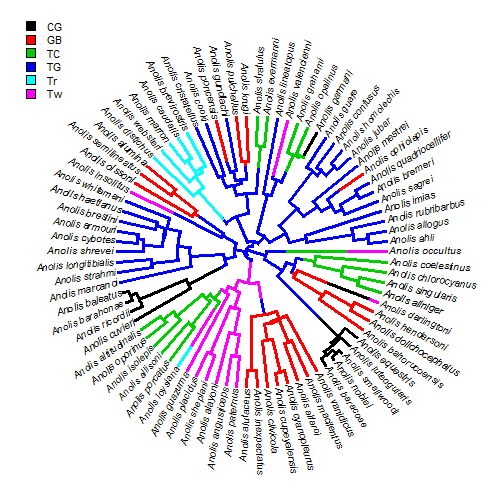``````## -- Normally, we would be working with a set of stochastic character
## -- histories, not just a single such history. Here's an example in which
## -- we first do stochastic mapping, & then we plot the posterior
## -- probabilities that each node is in each state.

ecomorph<-as.factor(getStates(anoletree,"tips"))
trees<-make.simmap(anoletree,ecomorph,model="ER",nsim=100)
``````
``````## make.simmap is sampling character histories conditioned on the transition matrix
##
## Q =
##             CG          GB          TC          TG          Tr          Tw
## CG -0.11570723  0.02314145  0.02314145  0.02314145  0.02314145  0.02314145
## GB  0.02314145 -0.11570723  0.02314145  0.02314145  0.02314145  0.02314145
## TC  0.02314145  0.02314145 -0.11570723  0.02314145  0.02314145  0.02314145
## TG  0.02314145  0.02314145  0.02314145 -0.11570723  0.02314145  0.02314145
## Tr  0.02314145  0.02314145  0.02314145  0.02314145 -0.11570723  0.02314145
## Tw  0.02314145  0.02314145  0.02314145  0.02314145  0.02314145 -0.11570723
## (estimated using likelihood);
## and (mean) root node prior probabilities
## pi =
##        CG        GB        TC        TG        Tr        Tw
## 0.1666667 0.1666667 0.1666667 0.1666667 0.1666667 0.1666667
``````
``````## Done.
``````
``````obj<-summary(trees,plot=FALSE)
plot(obj,colors=cols,type="fan",fsize=0.8,cex=c(0.5,0.3))
y=0.9*par()\$usr,prompt=FALSE,fsize=0.9)
``````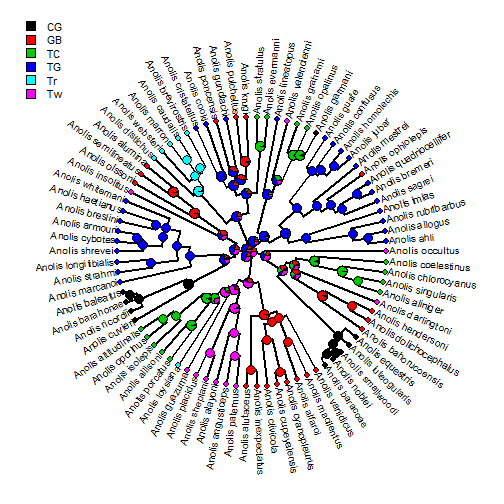``````## -- These are not visualizations for phylogenies, per se, but there are
## -- several other related plotting methods in the phytools that we can
## -- employ here. For instance, we can visualize our fitted discrete
## -- character model:

fit.ARD<-fitMk(anoletree,ecomorph,model="ARD")
plot(fit.ARD,main="fitted ARD model for ecomorph evolution")
``````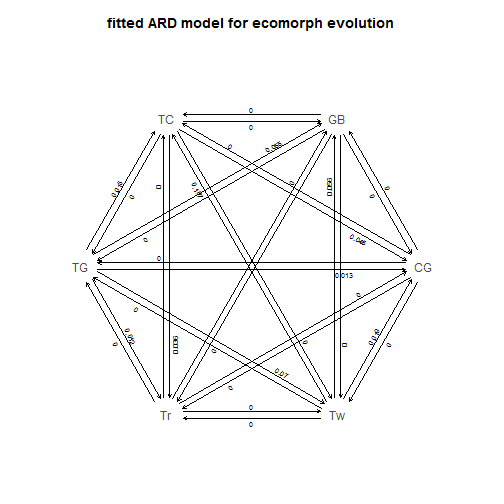``````plot(fit.ARD,show.zeros=FALSE,main="fitted ARD model for ecomorph evolution")
``````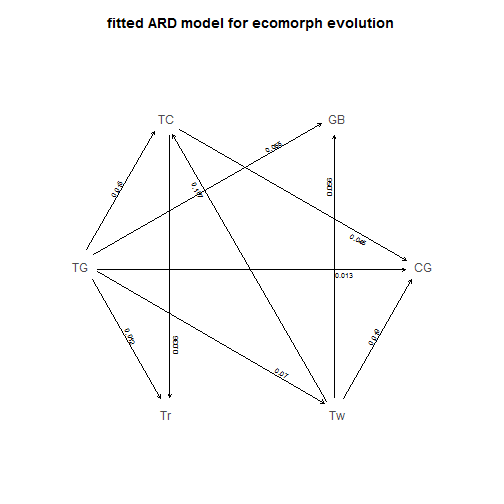``````## -- We can also visualize the posterior density of changes of different types
## -- on the tree. For example:

dd<-density(trees)
plot(dd)
``````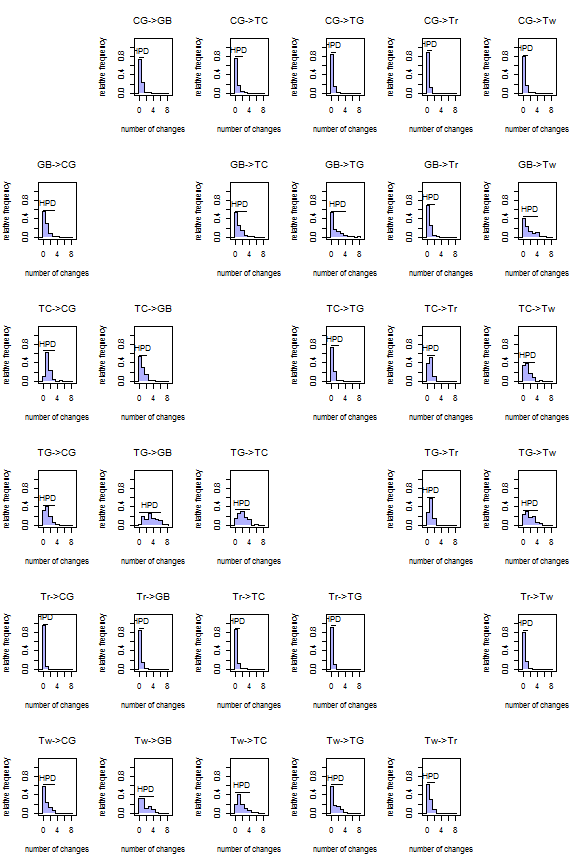``````## -- For binary traits we have more options still. In this example we will
## -- use a different empirical dataset consisting of feeding mode (biting vs.
## -- suction feeding) in elomorph eels. The first plot is just the character
## -- distribution at the tips; whereas the second is the posterior density
## -- from stochastic mapping at the edges & nodes of the tree.

fmode<-as.factor(setNames(eel.data[,1],rownames(eel.data)))
dotTree(eel.tree,fmode,colors=setNames(c("blue","red"),
c("suction","bite")),ftype="i",fsize=0.7)
``````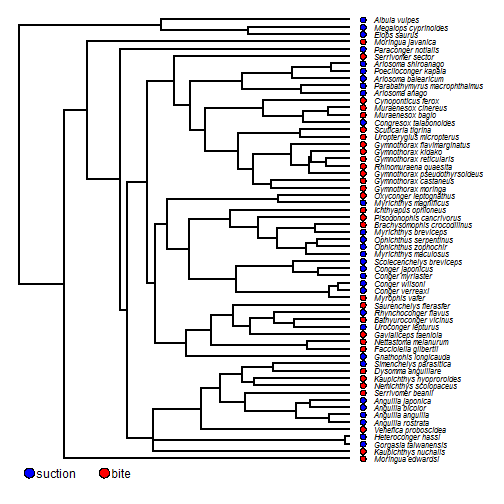``````eel.trees<-make.simmap(eel.tree,fmode,nsim=100)
``````
``````## make.simmap is sampling character histories conditioned on the transition matrix
##
## Q =
##                bite     suction
## bite    -0.01582783  0.01582783
## suction  0.01582783 -0.01582783
## (estimated using likelihood);
## and (mean) root node prior probabilities
## pi =
##    bite suction
##     0.5     0.5
``````
``````## Done.
``````
``````obj<-densityMap(eel.trees,states=c("suction","bite"),plot=FALSE)
``````
``````## sorry - this might take a while; please be patient
``````
``````plot(obj,lwd=4,outline=TRUE,fsize=c(0.7,0.9),legend=50)
``````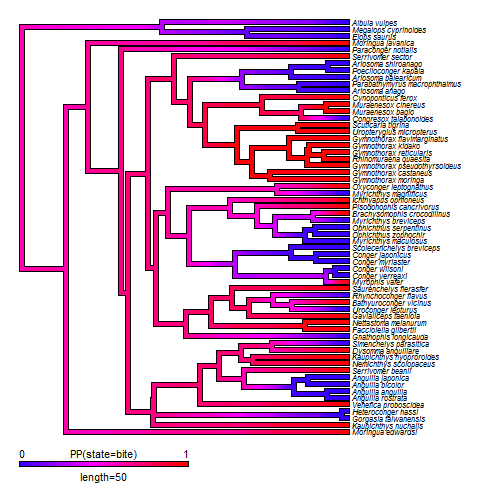``````## -- Or we can show the full posterior sample of changes of each type on the
## -- tree. These are not changes from any single stochastic character history,
## -- but changes in our full posterior sample of such histories.

dotTree(eel.tree,fmode,colors=setNames(c("red","blue"),c("bite","suction")),
fsize=0.7,ftype="i",legend=FALSE)
"suction")),prompt=FALSE,vertical=FALSE,shape="circle")
nulo<-sapply(eel.trees,markChanges,sapply(setNames(c("red","blue"),
c("bite","suction")),make.transparent,0.05))
c("bite","suction"))[2:1],
c("bite->suction","suction->bite")),
make.transparent,0.1),prompt=FALSE,x=50,y=-4,vertical=FALSE)
``````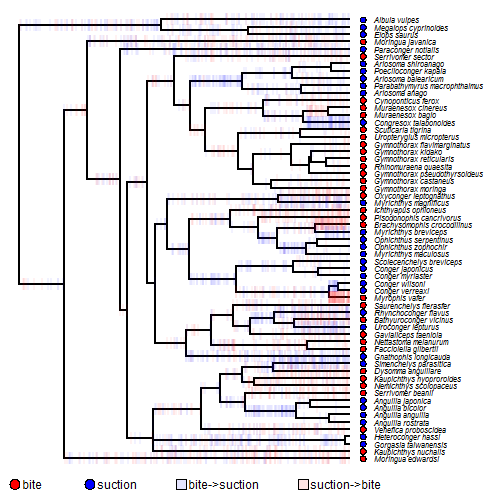``````## -- In addition to these methods for either continuous or discretely
## -- measured character traits, we can easily combine the methods.

## -- For instance, in the following I will overlay the posterior density
## -- from stochastic mapping on a dotTree plot of the continuous trait of
## -- overall body size.

bsize<-setNames(eel.data[,2],rownames(eel.data))
dotTree(eel.tree,bsize,length=10,fsize=0.7,lwd=7,ftype="i")
text(x=28,y=-1.8,"body size (cm)",pos=4)
xlim=get("last_plot.phylo",envir=.PlotPhyloEnv)\$x.lim,
ylim=get("last_plot.phylo",envir=.PlotPhyloEnv)\$y.lim)
prompt=FALSE,x=70,y=-2)
``````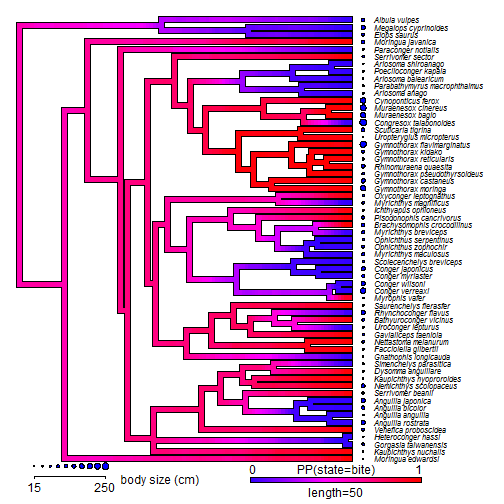``````## -- Alternatively, we could do this as two facing plots - one of densityMap
## -- and the other of contMap styles:

layout(matrix(1:3,1,3),widths=c(0.39,0.22,0.39))
plot(obj,lwd=6,ftype="off",legend=60,outline=TRUE,fsize=c(0,1.2))
plot.new()
plot.window(xlim=c(-0.1,0.1),
ylim=get("last_plot.phylo",envir=.PlotPhyloEnv)\$y.lim)
par(cex=0.6)
text(rep(0,length(obj\$tree\$tip.label)),1:Ntip(obj\$tree),
gsub("_"," ",obj\$tree\$tip.label),font=3)
plot(setMap(contMap(eel.tree,bsize,plot=FALSE),invert=TRUE),lwd=6,outline=TRUE,
direction="leftwards",ftype="off",legend=60,fsize=c(0,1.2),
leg.txt="body size (cm)")
``````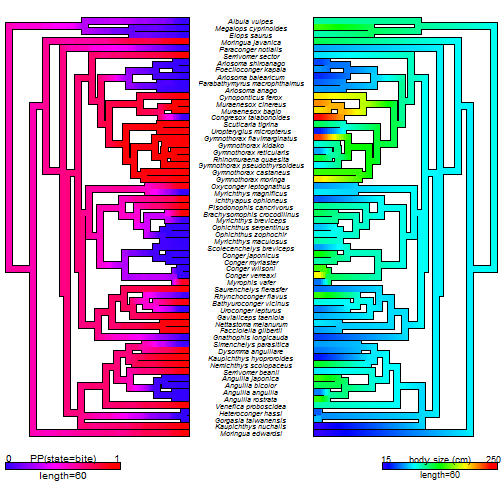``````## -- As another example here is a stochastically mapped discrete character
## -- history overlain on a traitgram plot of body size. At nodes I will add
## -- the posterior probabilties from stochastic mapping.

phenogram(eel.trees[],bsize,lwd=3,colors=
setNames(c("blue","red"),c("suction","bite")),
ftype="i")
``````
``````## Optimizing the positions of the tip labels...
``````
``````add.simmap.legend(colors=setNames(c("blue","red"),c("suction","bite")),
prompt=FALSE,shape="circle",x=0,y=250)
obj<-summary(eel.trees)
nodelabels(pie=obj\$ace,piecol=setNames(c("red","blue"),colnames(obj\$ace)),
cex=0.6)
tiplabels(pie=to.matrix(fmode[eel.tree\$tip.label],colnames(obj\$ace)),
piecol=setNames(c("red","blue"),colnames(obj\$ace)),cex=0.4)
``````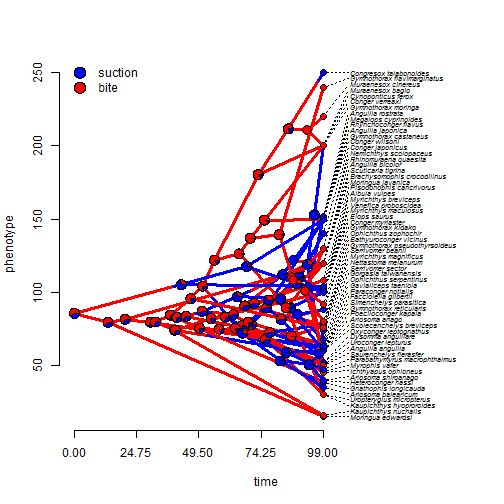``````## -- These are just a few examples. There are many other ways in which
## -- discrete & continuous character methods can be combined
``````
``````## -- I'm now going to demonstrate a handful of other phytools plotting methods.

## -- Firstly, phytools can be used to project a tree onto a geographic map.
## -- This can be done using linking lines from the tips of a square phylogram
## -- or directly.

obj<-phylo.to.map(tree,lat.long,plot=FALSE)
``````
``````## objective: 110
## objective: 110
## objective: 110
## objective: 110
## objective: 110
## objective: 110
## objective: 110
## objective: 110
``````
``````## objective: 100
## objective: 100
``````
``````## objective: 98
## objective: 98
## objective: 98
## objective: 98
## objective: 98
## objective: 98
``````
``````## objective: 96
``````
``````## objective: 92
## objective: 92
``````
``````## objective: 86
## objective: 86
## objective: 86
## objective: 86
## objective: 86
## objective: 86
``````
``````plot(obj,type="phylogram",asp=1.3,mar=c(0.1,0.5,3.1,0.1))
``````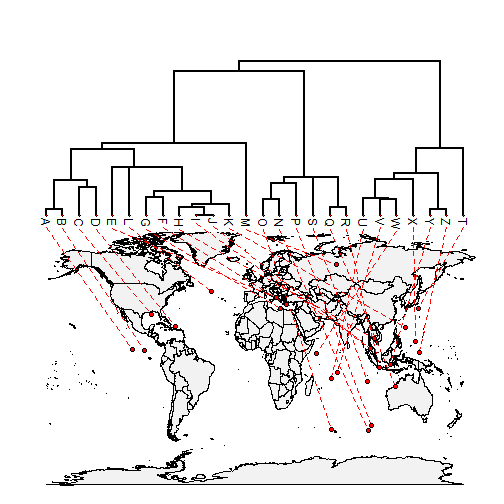``````plot(obj,type="direct",asp=1.3,mar=c(0.1,0.5,3.1,0.1))
``````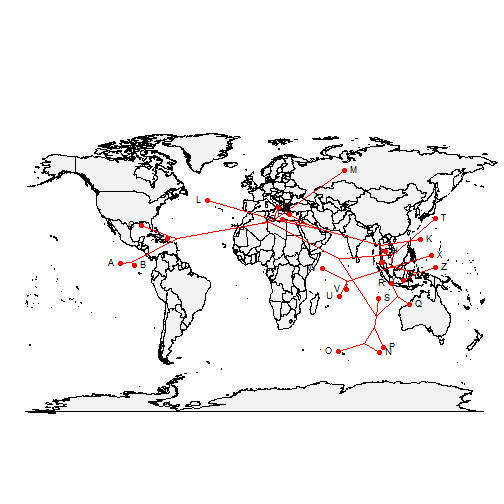``````## -- phytools can be used to create a co-phylogenetic plot in which two
## -- trees are graphed in a facing fashion, with linking lines between
## -- corresponding tips. The following uses data from Lopez-Vaamonde et al.
## -- (2001; MPE).

Pleistodontes<-trees[]
Sycoscapter<-trees[]
obj<-cophylo(Pleistodontes,Sycoscapter,assoc=assoc,
print=TRUE)
``````
``````## Rotating nodes to optimize matching...
``````
``````## objective: 359.577777777778
## objective: 359.577777777778
## objective: 359.577777777778
## objective: 359.577777777778
## objective: 359.577777777778
## objective: 359.577777777778
## objective: 359.577777777778
## objective: 359.577777777778
## objective: 359.577777777778
## objective: 359.577777777778
## objective: 359.577777777778
## objective: 359.577777777778
``````
``````## objective: 357.777777777778
## objective: 357.777777777778
``````
``````## objective: 345.111111111111
## objective: 345.111111111111
## objective: 345.111111111111
``````
``````## objective: 322.777777777778
``````
``````## objective: 201.17728531856
## objective: 201.17728531856
## objective: 201.17728531856
## objective: 201.17728531856
## objective: 201.17728531856
## objective: 201.17728531856
## objective: 201.17728531856
## objective: 201.17728531856
## objective: 201.17728531856
## objective: 201.17728531856
## objective: 201.17728531856
## objective: 201.17728531856
## objective: 201.17728531856
## objective: 201.17728531856
``````
``````## objective: 322.777777777778
## objective: 322.777777777778
## objective: 322.777777777778
## objective: 322.777777777778
## objective: 322.777777777778
## objective: 322.777777777778
## objective: 322.777777777778
## objective: 322.777777777778
## objective: 322.777777777778
## objective: 322.777777777778
## objective: 322.777777777778
## objective: 322.777777777778
## objective: 322.777777777778
## objective: 322.777777777778
## objective: 322.777777777778
## objective: 322.777777777778
## objective: 322.777777777778
## objective: 322.777777777778
``````
``````## objective: 201.17728531856
## objective: 201.17728531856
## objective: 201.17728531856
## objective: 201.17728531856
## objective: 201.17728531856
## objective: 201.17728531856
## objective: 201.17728531856
## objective: 201.17728531856
## objective: 201.17728531856
## objective: 201.17728531856
## objective: 201.17728531856
## objective: 201.17728531856
## objective: 201.17728531856
## objective: 201.17728531856
``````
``````## Done.
``````
``````plot(obj,link.type="curved",link.lwd=3,link.lty="solid",
``````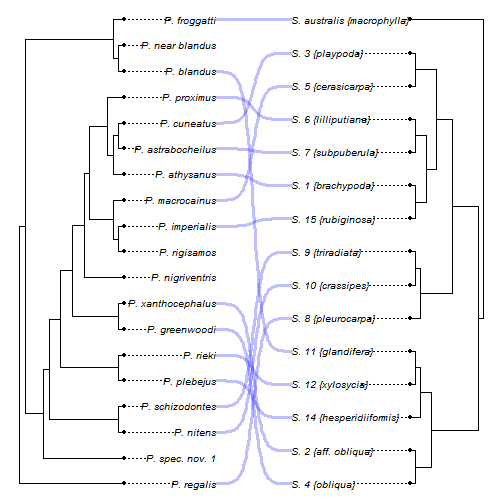``````## -- This method is fairly flexible. For instance, we can use add tip, node,
## -- or edge labels to each tree, or we can us different colors for different
## -- edges.

nodelabels.cophylo(which="left",frame="circle",cex=0.8)
nodelabels.cophylo(which="right",frame="circle",cex=0.8)
``````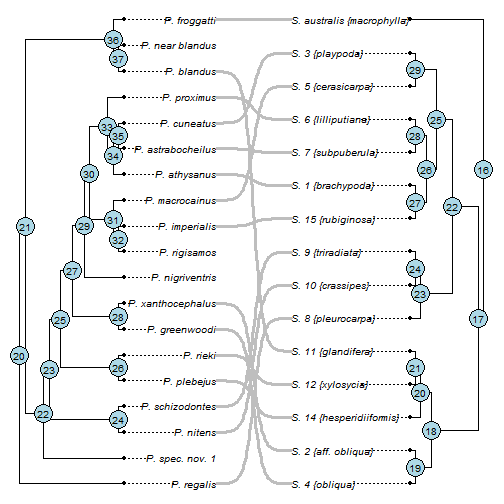``````left<-rep("black",nrow(obj\$trees[]\$edge))
nodes<-getDescendants(obj\$trees[],30)
left[sapply(nodes,function(x,y) which(y==x),y=obj\$trees[]\$edge[,2])]<-
"blue"
right<-rep("black",nrow(obj\$trees[]\$edge))
nodes<-getDescendants(obj\$trees[],25)
right[sapply(nodes,function(x,y) which(y==x),y=obj\$trees[]\$edge[,2])]<-
"blue"
edge.col<-list(left=left,right=right)
edge.col=edge.col)
``````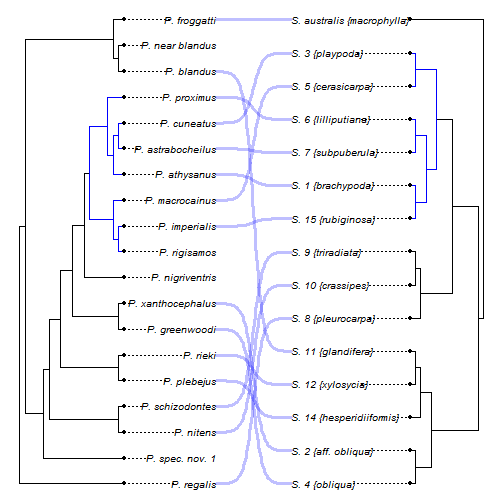``````## -- phytools contains a method for plotting a posterior sample of trees from
## -- Bayesian analysis. This is more or less equivalent to the stand-alone
## -- program densiTree. Here's a demo:

``````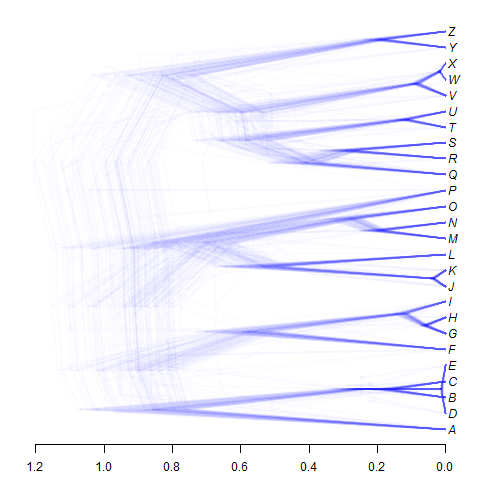``````## -- We can also just represent topological incongruence among trees:

densityTree(trees,use.edge.length=FALSE,type="phylogram",nodes="inner")
``````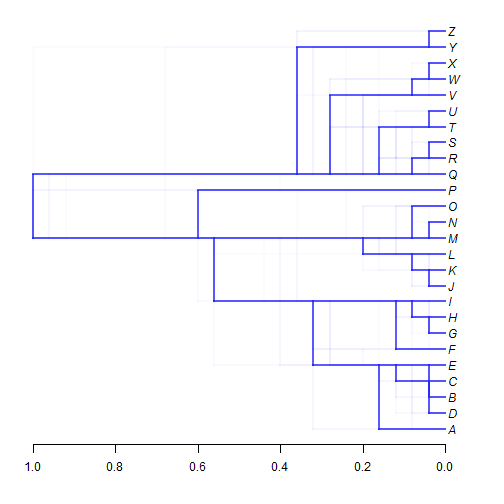``````## -- If we want to just compare two alternative time trees for the same set
## -- of taxa, we could try compare.chronograms. Here is a demo with simulated
## -- trees.

tree<-rtree(n=26,tip.label=LETTERS)
t1<-force.ultrametric(tree,"nnls")
tree\$edge.length<-runif(n=nrow(tree\$edge))
t2<-force.ultrametric(tree,"nnls")
compare.chronograms(t1,t2)
``````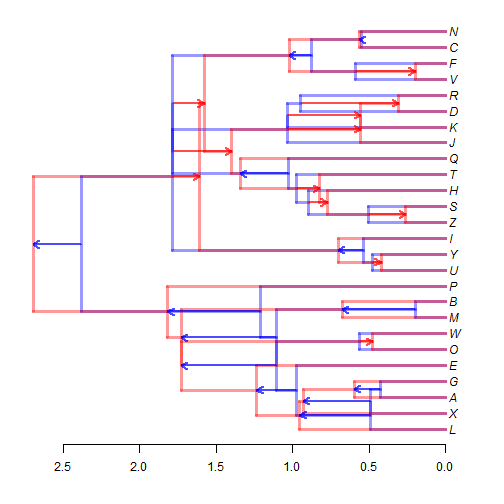``````## -- phytools has an interactive node labeling method. Here's a demo using a
## -- phylogeny of vertebrates (in non-interactive mode)

plotTree(vertebrates,xlim=c(-24,450))
labels<-c("Cartilaginous fish",
"Ray-finned fish",
"Lobe-finned fish",
"Anurans",
"Reptiles (& birds)",
"Birds",
"Mammals",
"Eutherians")
nodes<-labelnodes(text=labels,node=c(24,27,29,32,34,35,37,40),
shape="ellipse",cex=0.8,interactive=FALSE)
``````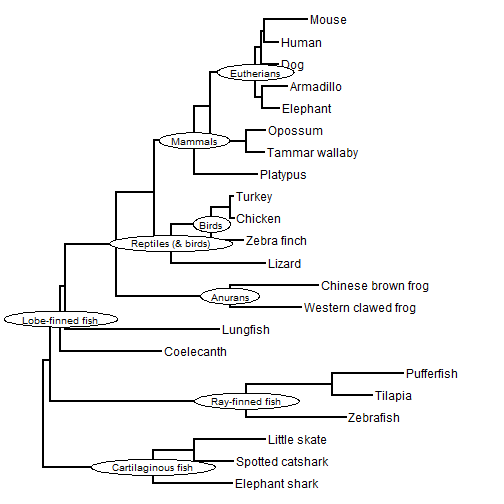``````## -- Another method in phytools allows us to easily label only a subset of tips
## -- in the tree. This can be handy for very large trees in which we are only
## -- interested in labeling a handful of taxa.

plot(anoletree,cols,xlim=c(0,9),ylim=c(-4,Ntip(anoletree)),ftype="off")